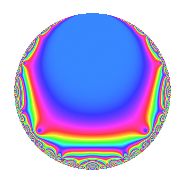# Properties

 Label 945.2.a.lLevel 945 Weight 2 Character orbit 945.a Self dual Yes Analytic conductor 7.546 Analytic rank 0 Dimension 2 CM No Inner twists 1

# Related objects

## Newspace parameters

 Level: $$N$$ = $$945 = 3^{3} \cdot 5 \cdot 7$$ Weight: $$k$$ = $$2$$ Character orbit: $$[\chi]$$ = 945.a (trivial)

## Newform invariants

 Self dual: Yes Analytic conductor: $$7.54586299101$$ Analytic rank: $$0$$ Dimension: $$2$$ Coefficient field: $$\Q(\sqrt{5})$$ Coefficient ring: $$\Z[a_1, a_2]$$ Coefficient ring index: $$1$$ Fricke sign: $$-1$$ Sato-Tate group: $\mathrm{SU}(2)$

## $q$-expansion

Coefficients of the $$q$$-expansion are expressed in terms of $$\beta = \frac{1}{2}(1 + \sqrt{5})$$. We also show the integral $$q$$-expansion of the trace form.

 $$f(q)$$ $$=$$ $$q + ( 1 + \beta ) q^{2} + 3 \beta q^{4} - q^{5} + q^{7} + ( 1 + 4 \beta ) q^{8} +O(q^{10})$$ $$q + ( 1 + \beta ) q^{2} + 3 \beta q^{4} - q^{5} + q^{7} + ( 1 + 4 \beta ) q^{8} + ( -1 - \beta ) q^{10} + ( 3 - 2 \beta ) q^{11} + ( -2 + \beta ) q^{13} + ( 1 + \beta ) q^{14} + ( 5 + 3 \beta ) q^{16} + ( 1 + 3 \beta ) q^{17} + ( -2 - \beta ) q^{19} -3 \beta q^{20} + ( 1 - \beta ) q^{22} + ( 4 + 3 \beta ) q^{23} + q^{25} - q^{26} + 3 \beta q^{28} + ( 4 - 3 \beta ) q^{29} + ( -1 - 4 \beta ) q^{31} + ( 6 + 3 \beta ) q^{32} + ( 4 + 7 \beta ) q^{34} - q^{35} + ( 3 - 8 \beta ) q^{37} + ( -3 - 4 \beta ) q^{38} + ( -1 - 4 \beta ) q^{40} + ( 7 - 9 \beta ) q^{41} + ( -1 + 2 \beta ) q^{43} + ( -6 + 3 \beta ) q^{44} + ( 7 + 10 \beta ) q^{46} + ( 3 - 4 \beta ) q^{47} + q^{49} + ( 1 + \beta ) q^{50} + ( 3 - 3 \beta ) q^{52} + ( 5 - 5 \beta ) q^{53} + ( -3 + 2 \beta ) q^{55} + ( 1 + 4 \beta ) q^{56} + ( 1 - 2 \beta ) q^{58} + ( 1 - 8 \beta ) q^{59} + ( -2 - 3 \beta ) q^{61} + ( -5 - 9 \beta ) q^{62} + ( -1 + 6 \beta ) q^{64} + ( 2 - \beta ) q^{65} + ( -7 - 3 \beta ) q^{67} + ( 9 + 12 \beta ) q^{68} + ( -1 - \beta ) q^{70} + ( 6 + 5 \beta ) q^{71} + ( -3 + 8 \beta ) q^{73} + ( -5 - 13 \beta ) q^{74} + ( -3 - 9 \beta ) q^{76} + ( 3 - 2 \beta ) q^{77} + ( -3 + 11 \beta ) q^{79} + ( -5 - 3 \beta ) q^{80} + ( -2 - 11 \beta ) q^{82} + ( 11 - 2 \beta ) q^{83} + ( -1 - 3 \beta ) q^{85} + ( 1 + 3 \beta ) q^{86} + ( -5 + 2 \beta ) q^{88} + ( -5 + 6 \beta ) q^{89} + ( -2 + \beta ) q^{91} + ( 9 + 21 \beta ) q^{92} + ( -1 - 5 \beta ) q^{94} + ( 2 + \beta ) q^{95} + ( 5 - 5 \beta ) q^{97} + ( 1 + \beta ) q^{98} +O(q^{100})$$ $$\operatorname{Tr}(f)(q)$$ $$=$$ $$2q + 3q^{2} + 3q^{4} - 2q^{5} + 2q^{7} + 6q^{8} + O(q^{10})$$ $$2q + 3q^{2} + 3q^{4} - 2q^{5} + 2q^{7} + 6q^{8} - 3q^{10} + 4q^{11} - 3q^{13} + 3q^{14} + 13q^{16} + 5q^{17} - 5q^{19} - 3q^{20} + q^{22} + 11q^{23} + 2q^{25} - 2q^{26} + 3q^{28} + 5q^{29} - 6q^{31} + 15q^{32} + 15q^{34} - 2q^{35} - 2q^{37} - 10q^{38} - 6q^{40} + 5q^{41} - 9q^{44} + 24q^{46} + 2q^{47} + 2q^{49} + 3q^{50} + 3q^{52} + 5q^{53} - 4q^{55} + 6q^{56} - 6q^{59} - 7q^{61} - 19q^{62} + 4q^{64} + 3q^{65} - 17q^{67} + 30q^{68} - 3q^{70} + 17q^{71} + 2q^{73} - 23q^{74} - 15q^{76} + 4q^{77} + 5q^{79} - 13q^{80} - 15q^{82} + 20q^{83} - 5q^{85} + 5q^{86} - 8q^{88} - 4q^{89} - 3q^{91} + 39q^{92} - 7q^{94} + 5q^{95} + 5q^{97} + 3q^{98} + O(q^{100})$$

## Embeddings

For each embedding $$\iota_m$$ of the coefficient field, the values $$\iota_m(a_n)$$ are shown below.

For more information on an embedded modular form you can click on its label.

Label $$\iota_m(\nu)$$ $$a_{2}$$ $$a_{3}$$ $$a_{4}$$ $$a_{5}$$ $$a_{6}$$ $$a_{7}$$ $$a_{8}$$ $$a_{9}$$ $$a_{10}$$
1.1
 −0.618034 1.61803
0.381966 0 −1.85410 −1.00000 0 1.00000 −1.47214 0 −0.381966
1.2 2.61803 0 4.85410 −1.00000 0 1.00000 7.47214 0 −2.61803
 $$n$$: e.g. 2-40 or 990-1000 Significant digits: Format: Complex embeddings Normalized embeddings Satake parameters Satake angles

## Inner twists

This newform does not admit any (nontrivial) inner twists.

## Atkin-Lehner signs

$$p$$ Sign
$$3$$ $$1$$
$$5$$ $$1$$
$$7$$ $$-1$$

## Hecke kernels

This newform can be constructed as the intersection of the kernels of the following linear operators acting on $$S_{2}^{\mathrm{new}}(\Gamma_0(945))$$:

 $$T_{2}^{2} - 3 T_{2} + 1$$ $$T_{11}^{2} - 4 T_{11} - 1$$